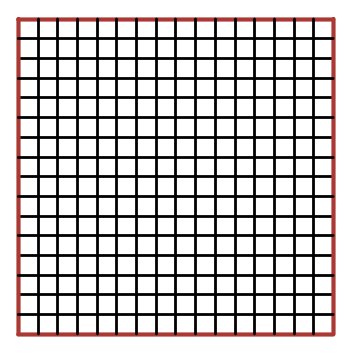# Red Perimeter 3 – Working Backwards

Probability Level 1If an $n \times n$ grid composed of unit squares has a red perimeter, and exactly 44 of the unit squares have exactly one red side, how long are the sides of the $n \times n$ square?

×